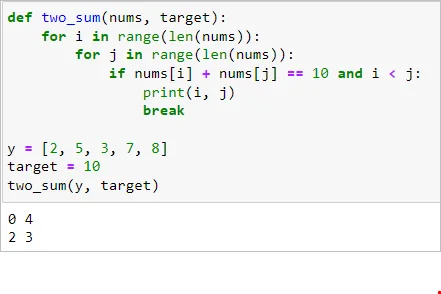28 May 2022

Posted on:

08 Jan 2022

1

# More than one sum in the list

what if i  have a list , my_list = [1,2,3,4,5,6,7,8,9] and my target is 10. here i know 6+4 = 10 , 9+1 = 10, 7+3=10,, and 8+2 = 10. when i run the code with this kind of list it only returns  index [3,5] which is equivalent to 4+6..
how can i solve a problem like this,

Posted on:

28 May 2022

0

Hi Abdulgafar,

I think something like this could work.

def two_sum(nums, target):
for i in range(len(nums)):
for j in range(len(nums)):
if nums[i] + nums[j] == 10 and i < j:
print(i, j)
break

y = [2, 5, 3, 7, 8]
target = 10
two_sum(y, target)